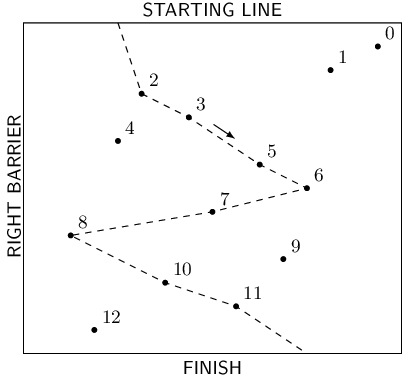### Solution to Slalom-Skiing by codility

https://codesays.com/2016/solution-to-slalom-skiing-by-codility/
You are a skier participating in a giant slalom. The slalom track is located on a ski slope, goes downhill and is fenced by barriers on both sides. The barriers are perpendicular to the starting line located at the top of the slope. There are N slalom gates on the track. Each gate is placed at a distinct distance from the starting line and from the barrier on the right-hand side (looking downhill).
You start from any place on the starting line, ski down the track passing as many gates as possible, and finish the slalom at the bottom of the slope. Passing a gate means skiing through the position of the gate.
You can ski downhill in either of two directions: to the left or to the right. When you ski to the left, you pass gates of increasing distances from the right barrier, and when you ski to the right, you pass gates of decreasing distances from the right barrier. You want to ski to the left at the beginning.
Unfortunately, changing direction (left to right or vice versa) is exhausting, so you have decided to change direction at most two times during your ride. Because of this, you have allowed yourself to miss some of the gates on the way down the slope. You would like to know the maximum number of gates that you can pass with at most two changes of direction.
The arrangement of the gates is given as an array A consisting of N integers, whose elements specify the positions of the gates: gate K (for 0 ≤ K < N) is at a distance of K+1 from the starting line, and at a distance of A[K] from the right barrier.
For example, consider array A such that:
A = 15 A = 13 A = 5 A = 7 A = 4 A = 10 A = 12 A = 8 A = 2 A = 11 A = 6 A = 9 A = 3The picture above illustrates the example track with N = 13 gates and a course that passes eight gates. After starting, you ski to the left (from your own perspective). You pass gates 2, 3, 5, 6 and then change direction to the right. After that you pass gates 7, 8 and then change direction to the left. Finally, you pass gates 10, 11 and finish the slalom. There is no possible way of passing more gates using at most two changes of direction.
Write a function:
int solution(int A[], int N);
that, given a zero-indexed array A consisting of N integers, describing the positions of the gates on the track, returns the maximum number of gates that you can pass during one ski run.
For example, given the above data, the function should return 8, as explained above.
For the following array A consisting of N = 2 elements:
A = 1 A = 5
the function should return 2.
Assume that:
• N is an integer within the range [1..100,000];
• each element of array A is an integer within the range [1..1,000,000,000];
• the elements of A are all distinct.
Complexity:
• expected worst-case time complexity is O(N*log(N));
• expected worst-case space complexity is O(N), beyond input storage (not counting the storage required for input arguments).
Elements of input arrays can be modified.

This is a wonderful variant of longest increasing subsequence. Because the longest increasing subsequence is a very classic question withe O(NlogN) solution, the key point to solve this question is to covert its original form to the classic question. We would like to extend it with two mirror universe.
The original question is convert into: find the longest increasing subsequence in the new extended multiverse. Here is an example of extended multiverse:
For each point P, it has three instances: the instance in the original world as P0, one in the mirror world as P1, and the last in the double-mirror world as P2. We process P2 firstly, then P1, and finally P0, so that to avoid the self-link sequence. In other words, we do not want that P2 is connected from P0 or P1, because they are essentially the same point.
The relationship between the original qualified subsequence (change direction at most two times) and the new longest increasing subsequence is 1:N mapping. Actually every subsequence in the original question has multiple equivalent subsequence in the new multiverse. Oppositely, every subsequence in the new multiverse has one equivalent in the original world.
def LongestIncreasingSubsequence(seq):
''' The classic dynamic programming solution for longest increasing
subsequence. More details could be found:
https://en.wikipedia.org/wiki/Longest_increasing_subsequence
http://www.geeksforgeeks.org/dynamic-programming-set-3-longest-increasing-subsequence/
http://stackoverflow.com/questions/3992697/longest-increasing-subsequence
'''
# smallest_end_value[i] = j means, for all i-length increasing
# subsequence, the minmum value of their last elements is j.
smallest_end_value = [None] * (len(seq) + 1)
# The first element (with index 0) is a filler and never used.
smallest_end_value = -1
# The length of the longest increasing subsequence.
lic_length = 0

for i in range(len(seq)):
# Binary search: we want the index j such that:
#     smallest_end_value[j-1] < seq[i]
#     AND
#     (  smallest_end_value[j] > seq[i]
#        OR
#        smallest_end_value[j] == None
#     )
# Here, the result "lower" is the index j.
lower = 0
upper = lic_length
while lower <= upper:
mid = (upper + lower) // 2
if seq[i] < smallest_end_value[mid]:
upper = mid - 1
elif seq[i] > smallest_end_value[mid]:
lower = mid + 1
else:
raise "Should never happen: " + \
"the elements of A are all distinct"

if smallest_end_value[lower] == None:
smallest_end_value[lower] = seq[i]
lic_length += 1
else:
smallest_end_value[lower] = \
min(smallest_end_value[lower], seq[i])

return lic_length

def solution(A):
# We are solving this question by creating two mirrors.
bound = max(A) + 1
multiverse = []
for point in A:
# The point in the double-mirror universe.
multiverse.append(bound * 2 + point)
# The point in the mirror universe.
multiverse.append(bound * 2 - point)
# The point in the original universe.
multiverse.append(point)

return LongestIncreasingSubsequence(multiverse)